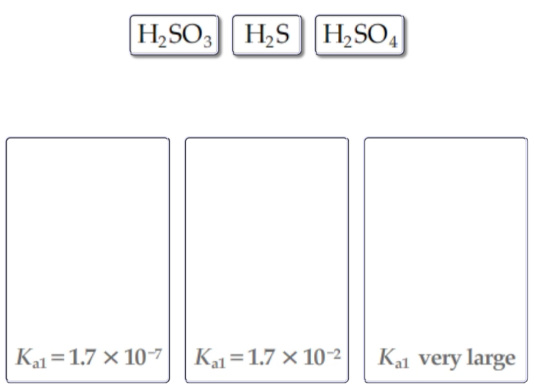# Rank the following compounds in order of decreasing acid strength using periodic trends

1) Rank the following compounds in order of decreasing acid strength using periodic trends.
Rank the acids from strongest to weakest. To rank items as equivalent, overlap them.
HBr, H2O, HCl, LiH

2) Without consulting the table of acid-dissociation constants, match the following acids to the given Ka1 values.

Drag the appropriate items to their respective bins.1)Srongest to weakest acids for the given set of acids is as below:

HBr > HCl > H2O > LiH

As the size increases in case of hydrogen halides, the acidic strength increases due to increase in size.

Hence HBr > HCl.

As we move along the period from left to right acidic strength increases.

In LiH, Li is more basic,it loses electrons easily and becomes Li+. H+ is not released as acid.

2)If the acid is strong then Ka value is very high. H2SO4 is the strong acid.

That indicates the conentration of H+ is high in the solution. Hence its Ka1 value is very large.

Among the given acids,H2S is weak acid, hence it has small value of Ka1.i.e., 1.7x10^-7

H2SO3-------------- Ka1 value is --------- 1.7x10^-2